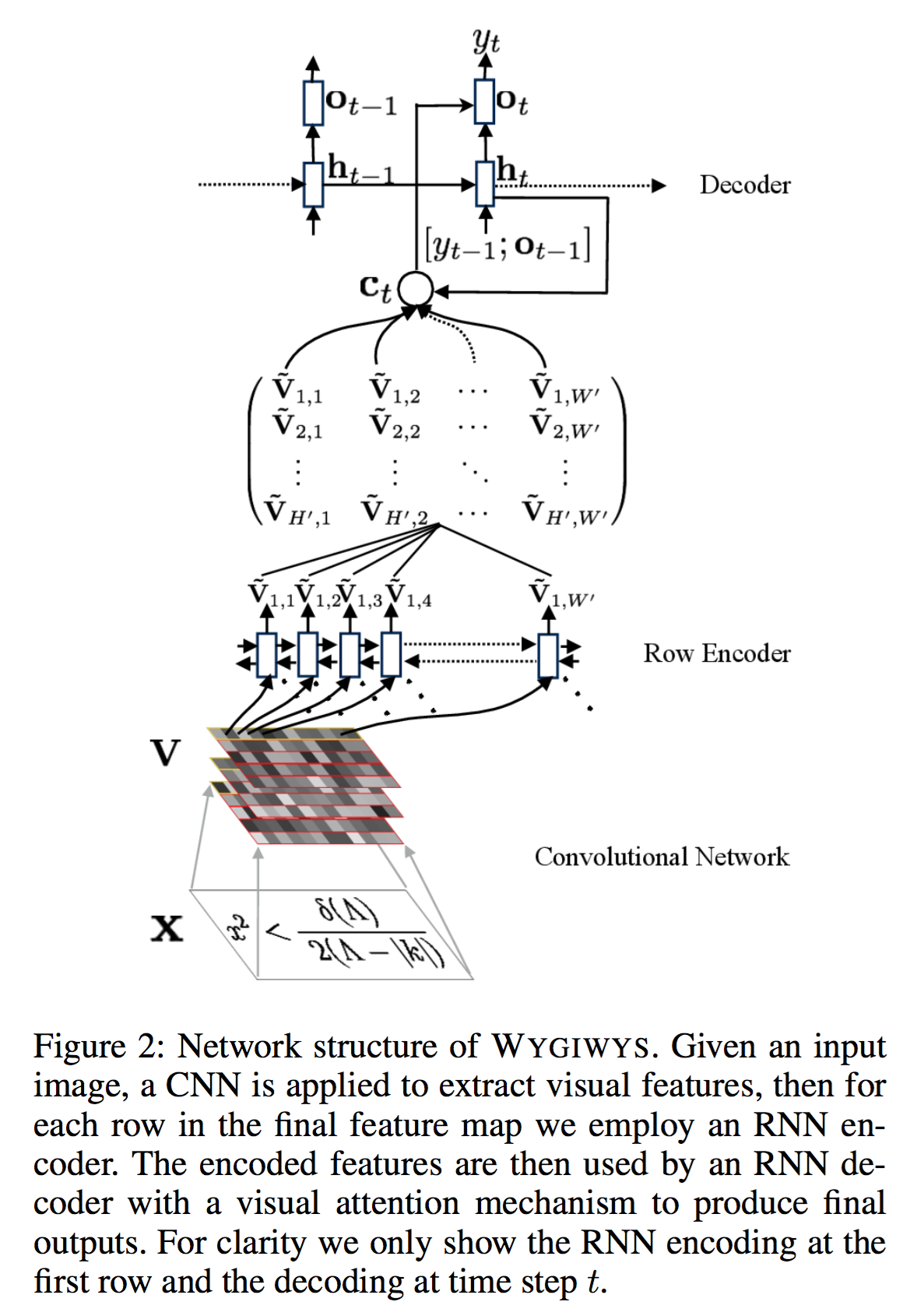What You Get Is What You See: A Visual Markup Decompiler

## 导言

OCR用来识别并提取结构信息：不仅仅要识别文字，还要提取语义。

The use of attention addi- tionally provides an alignment from the generated markup to the original source image

## Problem: Image-to-Markup Generation

• 图像：$x \in \mathcal{X}$，例如$\mathcal{X} = \mathbb{R}^{H \times W}$。
• 文本：$y = (y_1, y_2, ..., y_C); y \ in \mathcal{Y}, y_i \in \Sigma$。
• 编译：$\mathcal{Y} \rightarrow \mathcal{X}$.
• 需要学习一个反编译器！
• 训练：利用样本$(x, y)$训练学习一个反编译器。
• 测试：利用模型预测的$\hat{y}$和编译函数，生成一个图像$\hat{x}$，要求生成的图像和$x$一致。

## 模型 WYGIWYS• 图像特征抽取：CNN，没有全连接层，抽取的特征V尺寸为 $D \times H' \times W'$，分别是通道数，降维后的高度和宽度。
• 编码器：之前的ImageCaption不需要这个编码器，但是编码器可以学到顺序关系，这可以：
1. 学习 markup languages 的从左到右的顺序关系
2. 使用周围的上下文去编码隐层表达

• 解码器：优点复杂
1. 通过上述编码后的特征 grid $\tilde{V}$，加上历史隐层向量$h_{t-1}$学习一个注意力向量$\alpha_t$
2. 利用注意力向量和特征矩阵 $\tilde{V}$ 学习一个有注意力的上下文向量 $c_t$
3. 利用当前隐态向量 $h_t$ 和带有注意力的上下文 $c_t$ 学习一个输出向量 $o_t$，最终做softmax变换得到输出的词$y_t$！
4. 隐态更新采用常规的Decoder方案，即上一时刻的隐态$h_{t-1}$ 加上 上一时刻的输出 $o_t, y_{t-1}$